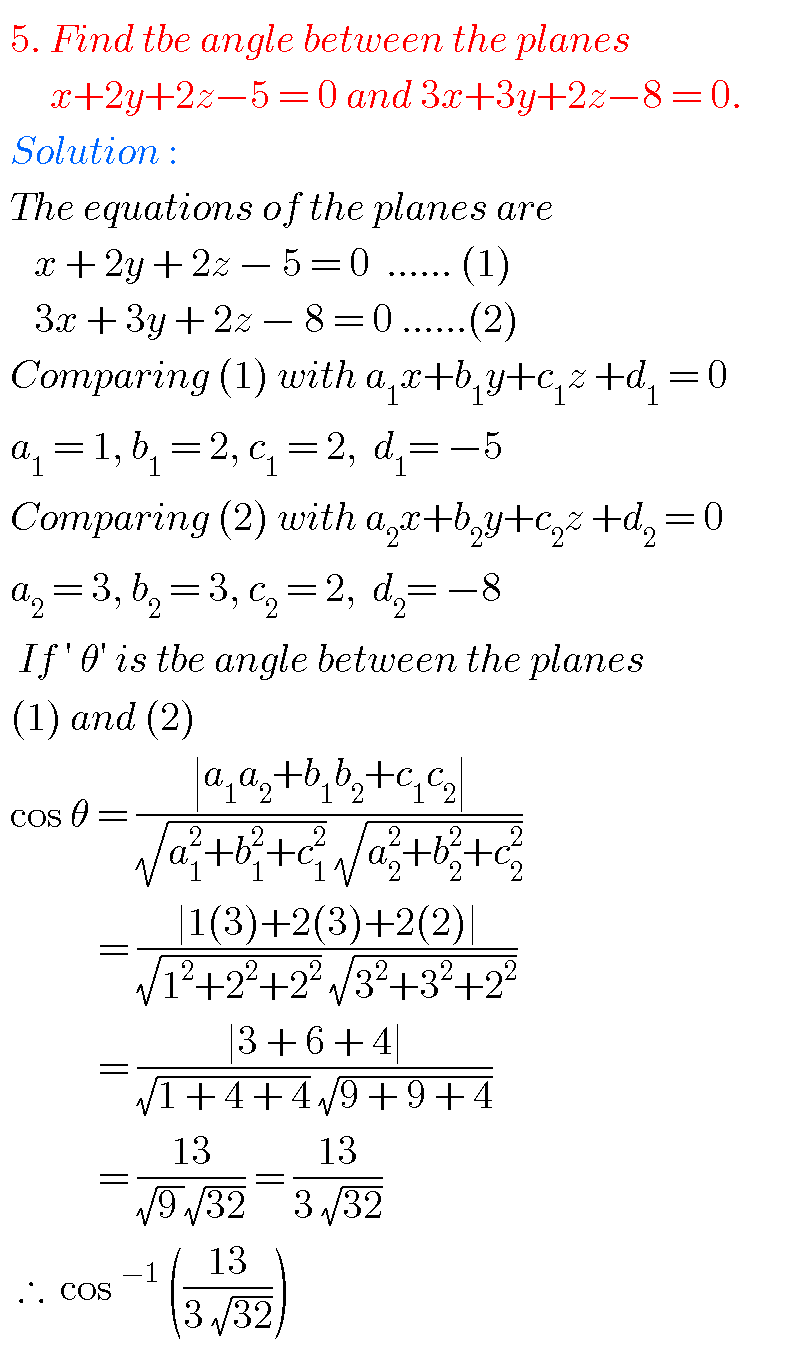## The plane,intermediate first year 1B mathematics chapter 7 problems with solutions,inter maths

THE PLANE, INTER FIRST YEAR MATHEMATICS 1B PROBLEMS WITH SOLUTIONS Mathematics 1B Intermediatesolutions for some problems are available here. These are very simple to understand. Study the textbook lesson The Plane very well. Observe the example problems and solutions given in the textbook Textbook solutions for The Plane Exercise 7(a) You can also see Inter …